# Halton Arp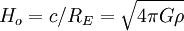,
where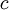is speed of light in vacuum,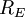is radius of curvature of space (called "Einstein's radius"),is Newtonian gravitational constant, and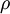is density of space  and so the cosmological redshift is exactly Arp's Intrinsic redshift with which one may measure the average density of universe (and possibly also the density of space around quasars).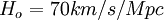implies